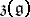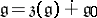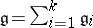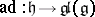# Lie algebra, reductive

A finite-dimensional Lie algebra over a fieldof characteristic 0 whose adjoint representation is completely reducible (cf. Adjoint representation of a Lie group; Representation of a Lie algebra). The property that a Lie algebrais reductive is equivalent to any of the following properties:

1) the radicalofcoincides with the centre;

2), whereis a semi-simple ideal of;

3), where theare prime ideals;

4)admits a faithful completely-reducible finite-dimensional linear representation.

The property that a Lie algebra is reductive is preserved by both extension and restriction of the ground field.

An important class of reductive Lie algebras overare the compact Lie algebras (see Lie group, compact). A Lie group with a reductive Lie algebra is often called a reductive Lie group. A Lie algebra overis reductive if and only if it is isomorphic to the Lie algebra of a reductive algebraic group over.

A generalization of the concept of a reductive Lie algebra is the following. A subalgebraof a finite-dimensional Lie algebraoveris said to be reductive inif the adjoint representationis completely reducible. In this caseis a reductive Lie algebra. Ifis algebraically closed, then for a subalgebraofto be reductive it is necessary and sufficient thatconsists of semi-simple linear transformations.